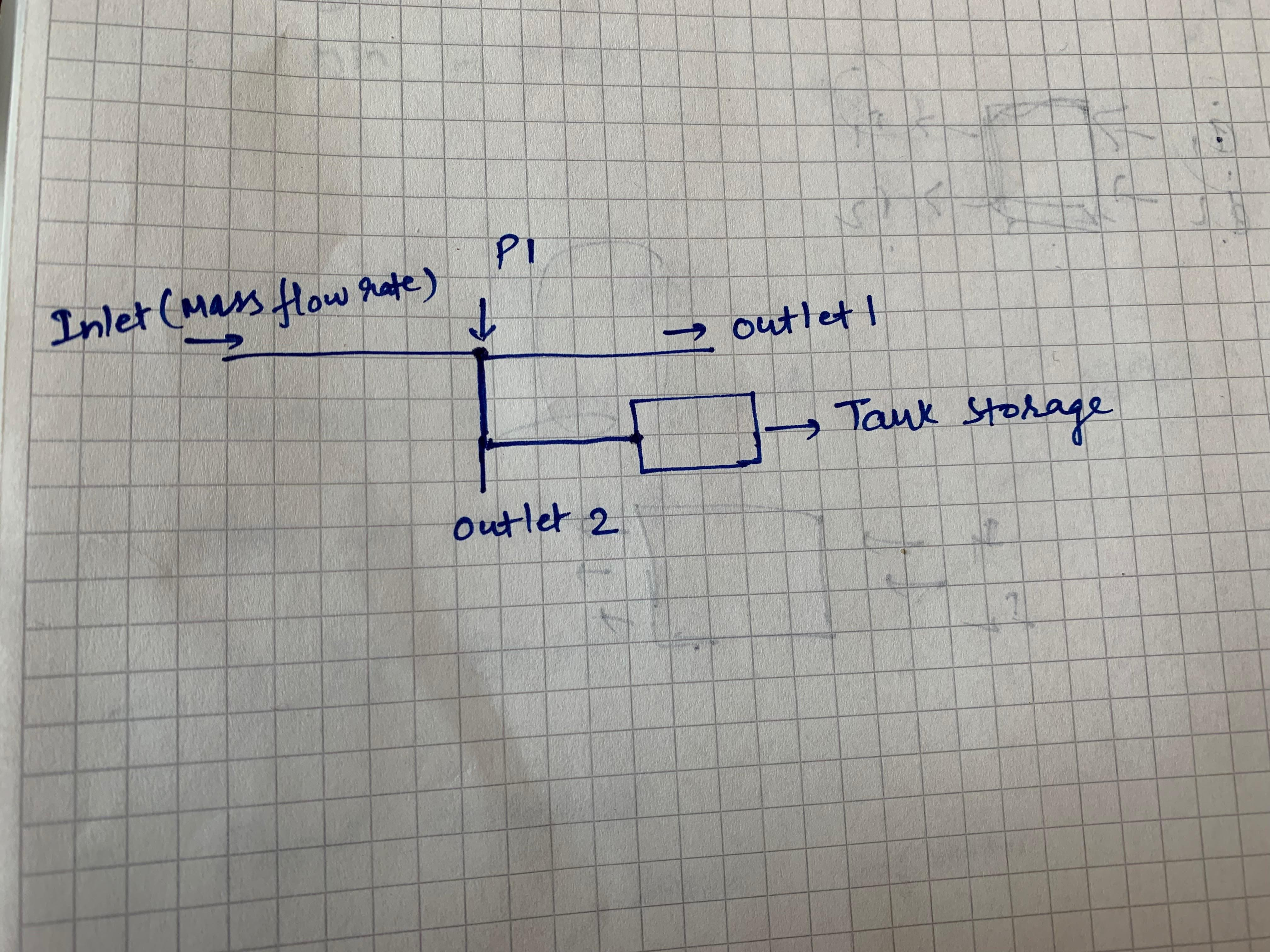## Fluids

•Rahul123
Subscriber

Hello

I am try to simulate a case for air flow, where my inlet boundary condition is mass flow rate. But, I am confused about correct boundary conditions for outlets.  I am attaching a figure of my setup here.

Flow from inlet should go and get stored in the tank in the figure. I also want to calculate pressure at point P1. And, outlet 1 and outlet 2 can be opened when desired. I tried by defining outlet 1 and 2 as pressure outlets. But, then the flow does not go to the tank at all. What is the correct boundary condition to achieve this?

Regards

Rahul

•DrAmine
Ansys Employee

•Rahul123
SubscriberThanks for the reply Amine, here is the setup. I want to setup a target mass flow rate as the parameters when I define outlets. So, basically I setup inflow mass rate at inlet and target mass flow rates at the both the outlets (which I defined as pressure outlets ) as the parameters

•Rob
Ansys Employee

Think through the physics:

what comes in (kg) = what goes out (kg) + hold-up (kg)

in steady state. Now consider the material density: how (why) will it fill the tank?

•Rahul123
Subscriber

Thanks for the reply. For ex: I defined my mass flow rate as 0.0004 kg/s as the input. My target mass flow rate in outlet 1 is 0.0001 and outlet 2 is 0.0001 as well. So, the rest of 0.0002 kg/s should fill in the tank. But, this not happening. Do I need to define an artificial boundary condition at the tank boundary to define the flow direction?

•Rob
Ansys Employee

Look at the material density: where does it go if you do that?  In a transient calculation as long as the material can go somewhere you can use mass in & out.

•Rahul123
Subscriber

My simulation is steady state. If I understood you correctly, you suggest me to use one more outlet 3 at the tank outer boundary. This makes it 3 outlets in total, out of which 2 outlets  (outlet 1 and 2) are real outlets same as the physical model, while outlet 3 is an artificial outlet defined only in the fluent model?

One more problem, I have is: I also want to measure pressure at point P1 as shown in the figure. I know my mass flow rate at inlet, but when I do that pressure at P1 comes much lower than the physical model. Any suggestion regarding that too?

Should I change the gauge pressure at outlets in order to achieve that? as, of now, I am using default gauge pressure (0) at both the outlets but with the target mass flow rate.

•DrAmine
Ansys Employee

You need to account for another degrees of freedoom: Transient accumatuon in the domain and / or compressiblity. Is the gauge pressure at both outlet the same?

•Rahul123
Subscriber

Yes, the gauge pressure is same at both the outlets but the target mass flow is different at both the outlets. I know my inlet mass flow rate, but it gives much lower pressure than experiment at P1. How can I resolve it? That fluid gets stored in the tank and I get the correct pressure at P1. I am using 101325 Pa as operating pressure.

Thanks!

regards

•DrAmine
Ansys Employee

If mass flow and total pressure inlet are known at the inlet then you need to decide which quantity is important for you. I do not think that you know the total pressure at inlet.

Are you now running transient? Are you now running compressible?

•Rahul123
Subscriber

Thanks for the reply Amine. I am running steady state simulation and I am using air flow, so yes it is compressible.

I know the mass flow at inlet from the physical experiments. The pressure at P1 as shown in the figure was measured in the experiment as well. When I use the same mass flow as experiments, the pressure at P1 is much lower. But, I guess I can fix that by defining the desired pressure at outlets? Would that be a correct approach?

And, how can I fix the problem of air not getting stored in the tank?

Thanks!

•DrAmine
Ansys Employee

So air is modeled as ideal gas?

Yes you fix that by increasing the pressure at outlet: but you said that here we have ambient conditions (surrounding). Or do you have any losses at the outlet which need to be accounted for?